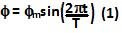## Sunday, March 29, 2009

### Irodov Problem 1.287As the cylinder spins about the axis XX' it has an angular velocity Iw directed along the axis XX' . Now the axis XX' undergoes oscillations (Irodov means simple harmonic oscillations even though he does not specifically mention it in the problem).

This means that the angle of orientationof the axis XX' at some instant of time ifs given,The angular momentum vector of the cylinder L, is thus, given by,This change in angular momentum is provided by the torque resulting from the force acting at the bearings given by F(l/2) + F(l/2) = Fl. Hence, we have,(see the list of moments of inertia)